[ Main Page | Research | Publications | Management | Grants | Industrial contracts | Courses | Conferences | Collaborators ]

# Feynman-Kac models and interacting particle systems

## A brief introduction to stochastic particle methods

This website is devoted to Feynman-Kac models and their interacting particle interpretation models. These stochastic models are increasingly used to sample from complex high-dimensional distributions. They approximate a given target probability distributions by a large cloud of random samples termed particles. Practically, the particles evolve randomly around the space independently and to each particle is associated a positive potential function. Periodically we duplicate particles with high potentials at the expense of particles with low potentials which die. This intuitive genetic mutation-selection type mechanism has appeared in numerous applications ranging from nonlinear filtering, Bayesian statistics, combinatorial counting, molecular and polymer simulation, rare events simulation, financial option pricing, quantum Monte Carlo methods, and genetic algorithms among others.

From a mathematical point of view, these methods can be interpreted as stochastic numerical approximations of Feynman-Kac measures. These measures represent the distribution of the paths of a reference Markov process, weighted by a collection of potential functions. These functional models are natural mathematical extensions of the traditional change of probability measures, commonly used in importance sampling. The particle interpretation consists in evolving a population of particles mimicking natural evolution mechanisms. During the mutation stage, the particles evolve independently of one another, according to the same probability transitions as the ones of the reference Markov chain. During the selection stage, each particle evaluates the potential value of its location. The ones with small relative values are killed, while the ones with high relative values are multiplied. The corresponding genealogical tree occupation measure converges, as the population size tends to infinity, to the complete Feynman-Kac distribution on path space.

The origins of stochastic particle simulation certainly starts with the seminal paper of N. Metropolis and S. Ulam: The Monte Carlo Method. Journal of the American Statistical Association, Vol. 44, No. 247, pp. 335-341 (1949). As explained by these two physicists in the introduction of their pioneering article, the Monte Carlo method is, "essentially, a statistical approach to the study of differential equations, or more generally, of integro-differential equations that occur in various branches of the natural sciences". The links between genetic type particle Monte Carlo models and quadratic type parabolic integro-differential equations has been developed in the beginning of 2000' in the series of articles on continuous time models
The earlier works on heuristic type genetic particle schemes seem to have started in Los Alamos National Labs with works of M.N. Rosenbluth and A.W. Rosenbluth [Monte-Carlo calculations of the average extension of macromolecular chains. J. Chem. Phys., 23:356-359, 1955], and T.E. Harris and H. Kahn. [Estimation of particle transmission by random sampling. Natl. Bur. Stand. Appl. Math. Ser., 12:27-30, 1951]. We also quote the work on artificial life of Nils Aall Barricelli at the Institute for Advanced Study in Princeton [Esempi numerici di processi di evoluzione, Methodos, pp. 45-68, 1954 & Symbiogenetic evolution processes realized by artificial methods, Methodos, Vol. 9:35-36, pp. 143-182, 1957]. In all of these works, the genetic Monte Carlo scheme is always presented as a natural heuristic resampling type algorithm to generate random population models, to sample molecular conformations, or to estimate high energy particle distributions, without a single convergence estimate to ensure the performance, nor the robustness of the Monte Carlo sampler.

Genetic type particle algorithms are now extensively and routinely used in engineering, statistics and physics under sometimes different names, such as :

Particle filters, bootstrap or genetic filters, population Monte Carlo methods, sequential Monte Carlo models, genetic search models, branching and multi-level splitting particle rare event simulations, condensation models, go-with-the winner, spawning models, walkers population reconfigurations, pruning-enrichment strategies, quantum and diffusion Monte Carlo, rejuvenation models, and many others.

The mathematical foundations, and the performance analysis of all of these discrete generation particle models are rather recent. The first rigorous study in this field seems to be the article published in 1996 on the applications of particle methods to nonlinear estimation problems :
This article provides the first proof of the unbiased property of particle likelihood approximation models (lemma 3 page 12); and adaptive resampling criteria w.r.t. the weight dispersions (see remark 1 on page p.4).

This article also presents the first convergence results for a new class of interacting particle filters, originally presented as heuristic Monte Carlo schemes in the beginning of the 1990's in three independent schools.
1. A series of classified industrial Research Contracts on tracking and control developped between 1990 and 1993 by the LAAS-CNRS Team : P. Del Moral, J.C. Noyer, G. Rigal, and G. Salut

2. The first journal article presenting the heuristic of particle filters

3. The first conference article presenting the heuristic of particle filters

The limiting Feynman-Kac probability measures discussed above are closely related to the so-called infinite population model arising in evolutionary computation literature. In this connection, we underline that the discrete prediction/updating filtering equation discussed in the articles on the convergence properties of particle filters1,2,3,5,6 as well as in the articles by Gordon-Salmon and Smith on the Bootstrap filter and the one by Kitagawa on the Monte Carlo filter coincide with the infinite population GA evolution equations discussed for instance by Ermakov and Zhiglyavskii in an article on GA published in 1984 (see equation(6)), as well as in the article by Qi and Palmeri (see equation (4)) and more recently in the article by Song and Li discussing issues and wrong convergence proofs in a widely cited studies on genetic algorithms in continuous space (see equation (1)). As mentioned earlier, Particle filters, Bootstrap filters as well as Monte Carlo filters also coincide with the Genetic algorithm with roulette-wheel selection.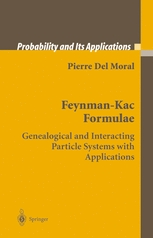For a more detailed description of the origins of particle methods, and their applications we refer to the following studies
1. Measure Valued Processes and Interacting Particle Systems. Application to Non Linear Filtering Problems.
Del Moral P., [ LSP-CNRS Research Report No. 96-15, Isbn 0248-3289 (1996) ] published in : Annals of Applied Probability 8 , no. 2, 438-495 (1998).

Look-ahead type strategies (section 4.2.2), reducing the variance using conditional explorations w.r.t. the observation sequences (example 3 p. 40), local errors transport models (proof of theo 1, p.11), mean field models w.r.t. the occupation measures of random trees (section 3.2).

2. Branching and Interacting Particle Systems Approximations of Feynman-Kac Formulae with Applications to Non-Linear Filtering.
Del Moral P., Miclo L., [ LSP-CNRS Research Report No. 99-05 (1999) ] published in : Séminaire de Probabilités XXXIV, Ed. J. Azéma and M. Emery and M. Ledoux and M. Yor. Lecture Notes in Mathematics, Springer-Verlag Berlin, Vol. 1729, 1-145 (2000).

3. Feynman-Kac formulae. Genealogical and interacting particle approximations Del Moral P., Springer New York, Series: Probability and Applications (2004).

Some stochastic models & methods discussed in this monograph :
Acceptance-rejection with recycling particle strategies, interacting Kalman filters a.k.a. Rao-Blackwellized particle filters (section 2.6, and section 12.6.7), look-ahead type strategies (section 12.6.6), genealogical tree models and branching strategies (section 11), interacting Metropolis-Hasting models (chapter 5), Dirichlet problems with boundary conditions (chapter 12), spectral analysis of Schrodinger operators (section 12.4), directed polymer simulation (section 12.5), rare event simulation (section 12.2.5, and section 12.3), smoothing algorithms (section 12.6).

and the more recent books

4. Pierre Del Moral, Peng Hu, Liming Wu
On the concentration properties of Interacting particle processes HAL-INRIA RR-7677 (2011),
Foundations and Trends in Machine Learning, Vol. 3, No. 3 - 4, 225-389 (2012). (online article, 167 pages)

5. P. Del Moral.
Mean field simulation for Monte Carlo integration.
Chapman & Hall/CRC Monographs on Statistics & Applied Probability [600p.] (to appear May 10th, 2013).The practitioner will find in the research book , and in the review article , a source of useful convergence estimates as well as a detailed list of concrete examples of particle approximations for real models, including restricted Markov chain simulations, random motions in absorbing media, spectral analysis of Schrodinger operators and Feynman-Kac semigroups, rare event analysis, sensitivity measure approximations, financial pricing numerical methods, parameter estimation in HMM models, island particle models, interacting MCMC models, statistical machine learning, bayesian inference, Dirichlet boundary problems, nonlinear filtering problems, interacting Kalman-Bucy filters, directed polymer simulations, stochastic optimisation, and interacting Metropolis type algorithms.

The recent book  presents the first comprehensive and modern mathematical treatment of these mean field particle models, including refined convergence analysis on nonlinear Markov chain models. It also covers applications related to parameter estimation in hidden Markov chain models, stochastic optimization, nonlinear filtering and multiple target tracking, stochastic optimization, calibration and uncertainty propagations in numerical codes, rare event simulation, financial mathematics, and free energy and quasi-invariant measures arising in computational physics and population biology. The book helps theoretical probability researchers, applied statisticians, biologists, statistical physicists, and computer scientists work better across their own disciplinary boundaries.

Monte Carlo and Mean Field Models. Theory and Applications. Feynman-Kac Models. Four Equivalent Particle Interpretations. Continuous-Time Feynman-Kac Models. Nonlinear Evolutions of Intensity Measures. Particle Absorption Models. Signal Processing and Control Systems. Mean Field Feynman-Kac Models. A General Class of Mean Field Models. Empirical Processes. Feynman-Kac Semigroups. Intensity Measure Semigroups. Particle Density Profiles. Genealogical Tree Models. Particle Normalizing Constants. Backward Particle Markov Models.

## Application domains

While the diversity of application model areas is part of the charm of the particle theory of Feynman-Kac models the list topics presented below is not exhaustive, and actually only reflects the tastes and interests of the author.
A series of recent tutorials presenting this interacting particle methodology, and some applications domains, is provided below :

• ## Software BiiPS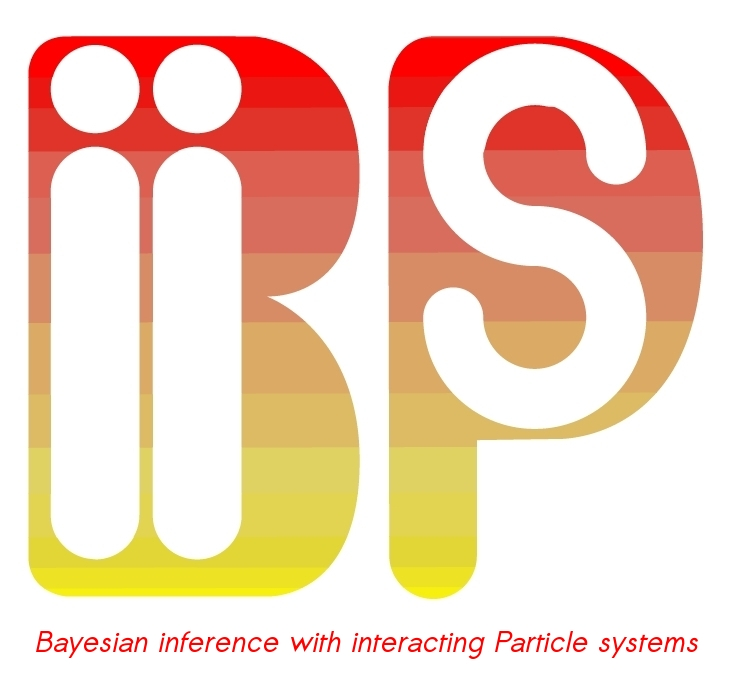The software BIIPS is a general software developed by the INRIA team ALEA for bayesian inference with interacting particle systems, a.k.a. Sequential Monte Carlo methods. A demonstration of the BiiPS software for estimating the stochastic volatility of financial data can be found in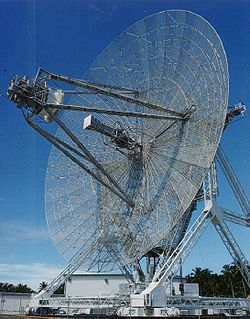### Filtering, Systems, Advanced Signal Processing

• Robotics
• Image Processing
• Audio signal processing
• Tracking &positioning
• GPS/INS Integration
• Fluids mechanics, forecasting, data assimilation,
• Optical tomography,
• Particle filters & likelihood estimation : Tutorials, surveys, methodology, performance analysis.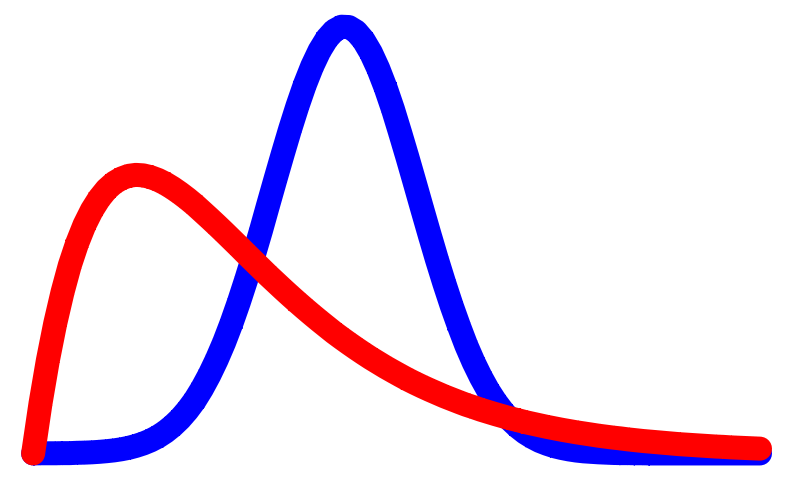### Bayesian Statistics

• Data Assimilation & Bayesian Analysis of Massive Datasets
• Spectral Bayesian Analysis
• Hidden Markov Models,
• Monte Carlo Smoothing for Nonlinear Time Series
• Open-loop regulation and tracking control### Financial Mathematics and Econometrics

• Recovering Volatility From Option Prices
• Business Cycle and Stock Market Volatility
• Cluster analysis of panels datasets
• Extracting Latent States from Assets Prices
• Portfolio Optimization under Partially Observed Stochastic Volatility
• Option Pricing using Multinomial Recombination Trees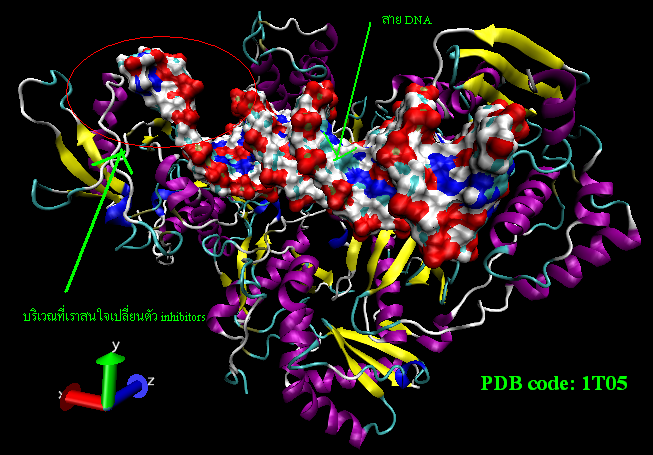### Biology and Computational Chemistry

• Particle Estimation of Schrodinger Ground States
• Particle Approach for Molecular Dynamics
• Macromolecular Chemistry and polymerization
• Complex Systems and lattice polymers
• Population dynamics and Bio-processes
• Genetic Mutation-Selection Balance
• Yaglom limits, Quasi-invariant measures, Feynman-Kac equilibrium measures
• Genealogical tree models and Interacting Jump Particle Systems### Rare events analysis

• Probabilistic aircraft conflict detection
• Small reachability probabilities for stochastic hybrid systems
• Collision risk in free flight air traffic
• Satellite versus debris collisions
• Fiber optics communications networks
• Financial risk analysis
• Adaptive multilevel splitting for rare event simulation
• Genealogical default trees, and particle analysis of rare events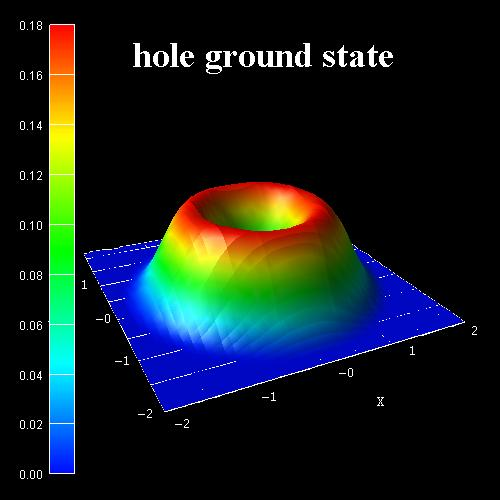### Applied Probability and Computational physics

• Molecular and Multiscale Modeling
• Probabilistic Interpretations Schrodinger operators
• Particle Motions in Absorbing Medium
• Ground States energy computations
• Equilibrium Sampling from Nonequilibrium
• Optical tomography,
• Complex turbulent systems
• Feynman-Kac-Schrodinger models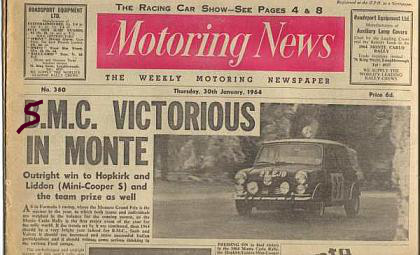• Sequential Monte Carlo methodology.
• Interacting Markov Chain Monte Carlo models.
• Particle Markov chain Monte Carlo methods.
• Gibbs cloner models, randomized Algorithms with Splitting
• Sequentially Interacting Markov Chain Monte Carlo models
• Mean field interacting particle models### Stochastic optimization, regulation, and optimal control

• Optimal Stopping times problems
• Maslov optimization theory
• Decision genealogical trees
• Sequential Monte Carlo algorithms
• Stochastic optimization
• Regulation of processes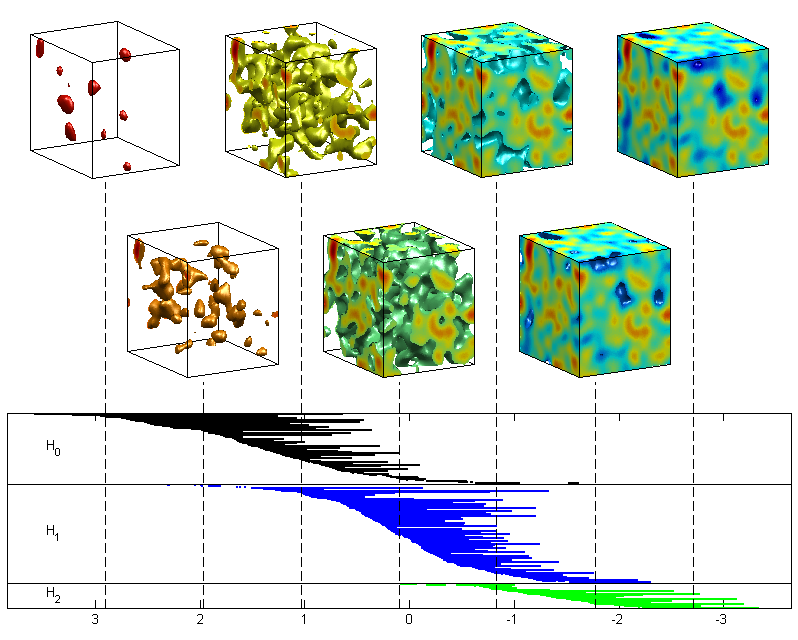### Measure Valued Processes, Stochastic PDE's

• Branching and Interacting particle approximations
• Interacting Markov Chain Monte Carlo Methods
• Stochastic models and stability analysis
• Feynman-Kac path integrals and semigroups
• Probabilistic Interpretations of Schrodinger operators
• Kushner-Stratonovitch Equation